# Koch Curve or Koch Snowflake

What is Koch Curve?

The Koch snowflake (also known as the Koch curve, Koch star, or Koch island) is a mathematical curve and one of the earliest fractal curves to have been described. It is based on the Koch curve, which appeared in a 1904 paper titled “On a continuous curve without tangents, constructible from elementary geometry” by the Swedish mathematician Helge von Koch.

The progression for the area of the snowflake converges to 8/5 times the area of the original triangle, while the progression for the snowflake’s perimeter diverges to infinity. Consequently, the snowflake has a finite area bounded by an infinitely long line.

# Construction

Step1:

Draw an equilateral triangle. You can draw it with a compass or protractor, or just eyeball it if you don’t want to spend too much time drawing the snowflake.

• It’s best if the length of the sides are divisible by 3, because of the nature of this fractal. This will become clear in the next few steps.
•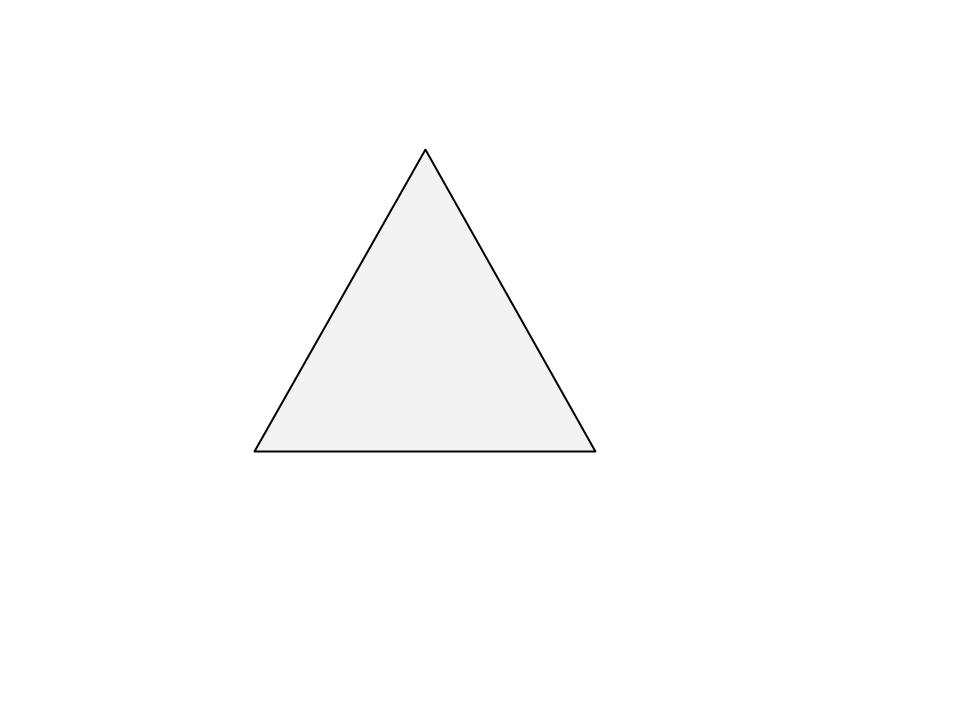Step2:

Divide each side in three equal parts. This is why it is handy to have the sides divisible by three.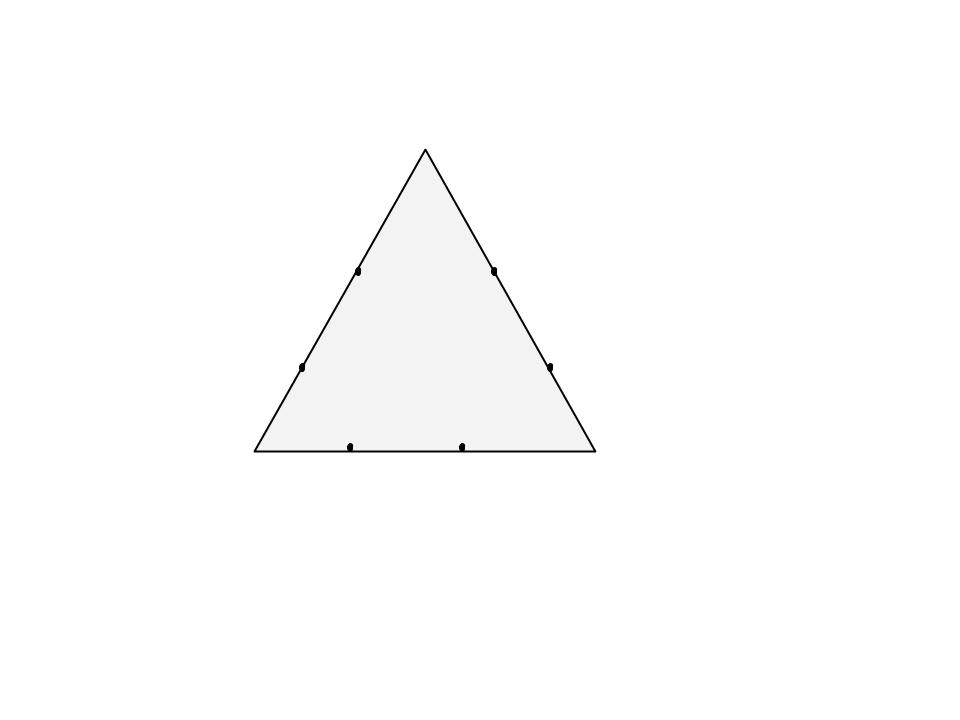Step3:

Draw an equilateral triangle on each middle part. Measure the length of the middle third to know the length of the sides of these new triangles.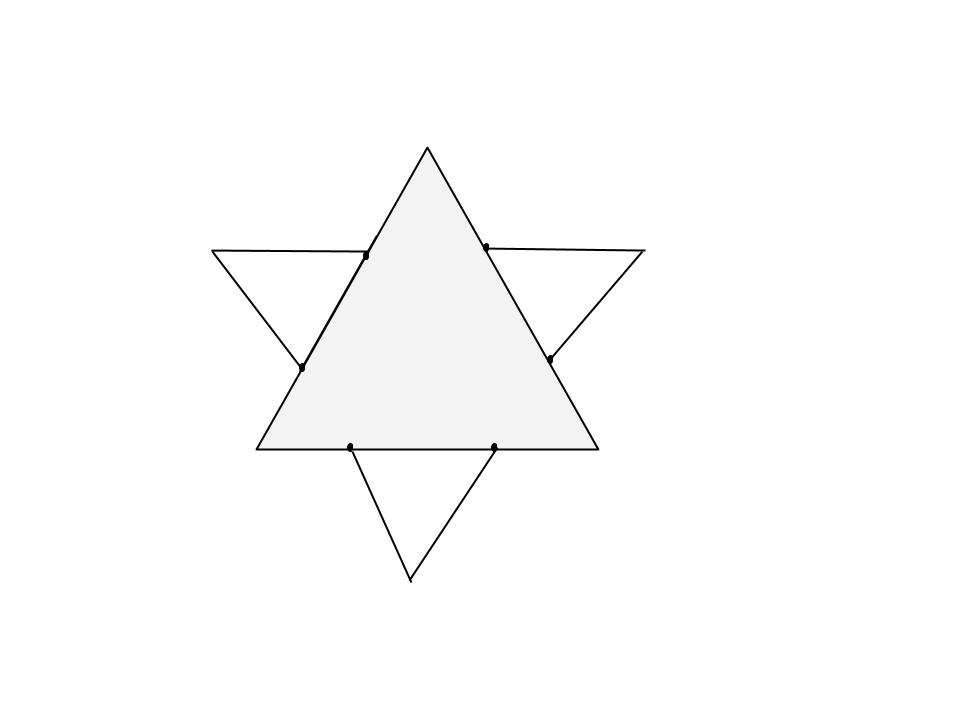Step4:

Divide each outer side into thirds. You can see the 2nd generation of triangles covers a bit of the first. These three line segments shouldn’t be parted in three.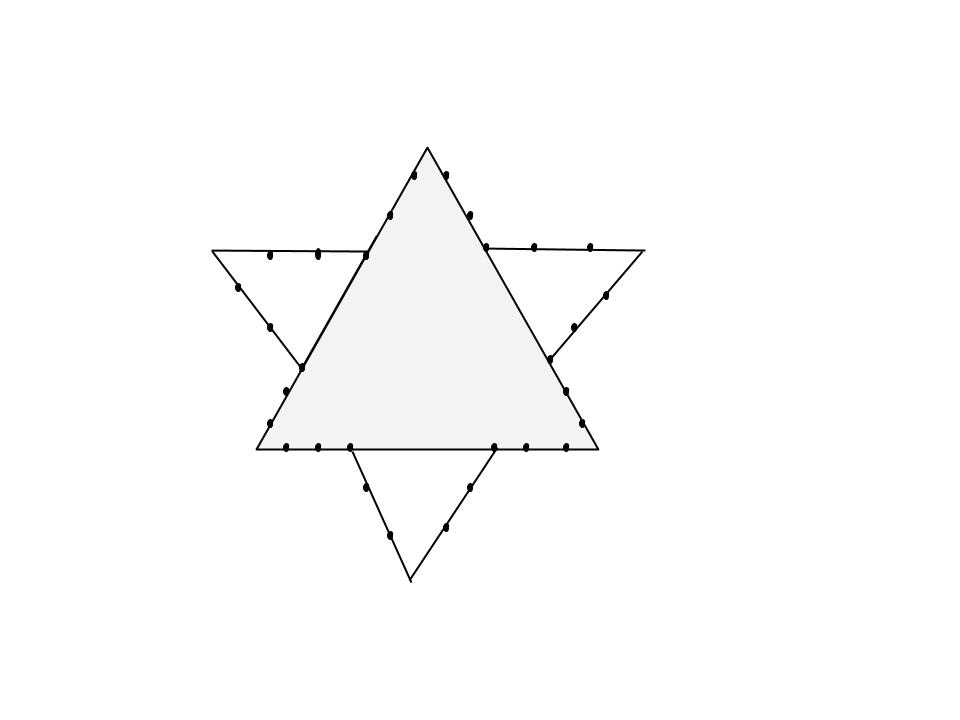Step5:

Draw an equilateral triangle on each middle part.

• Note how you draw each next generation of parts that are one 3rd of the mast one.
•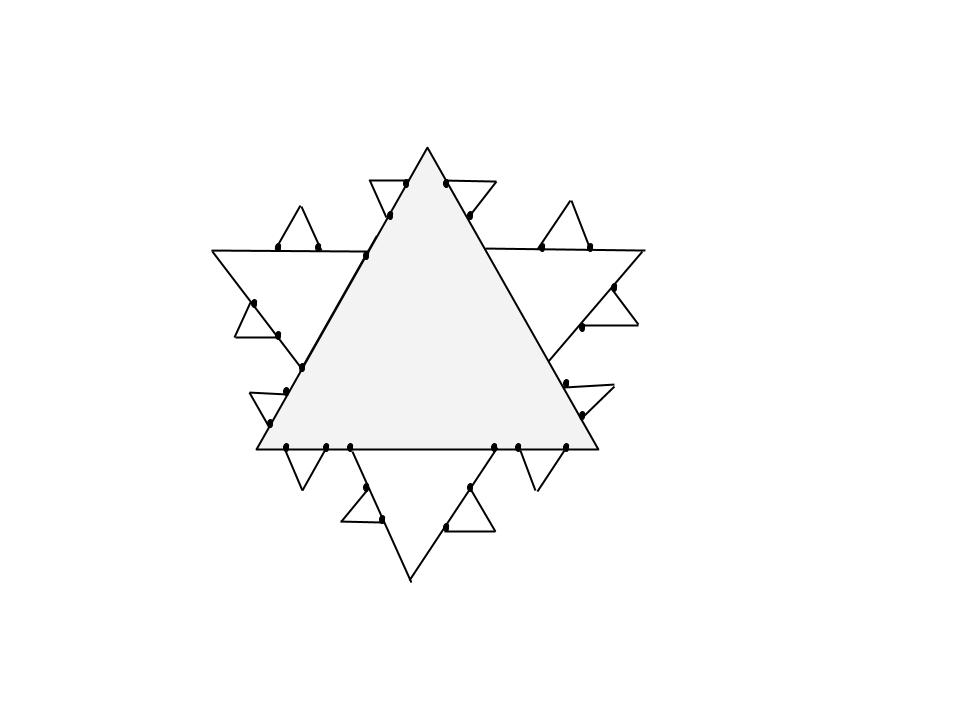# Representation as Lindenmayer system

The Koch curve can be expressed by the following rewrite system (Lindenmayer system):

Alphabet : F
Constants : +, ?
Axiom : F
Production rules: F ? F+F–F+F

Here, F means “draw forward”, – means “turn right 60°”, and + means “turn left 60°”.
To create the Koch snowflake, one would use F++F++F (an equilateral triangle) as the axiom.

# To create a Koch Curve :

 `# Python program to print partial Koch Curve. ` `# importing the libraries : turtle standard  ` `# graphics library for python ` `from` `turtle ``import` `*` ` `  `#function to create koch snowflake or koch curve ` `def` `snowflake(lengthSide, levels): ` `    ``if` `levels ``=``=` `0``: ` `        ``forward(lengthSide) ` `        ``return` `    ``lengthSide ``/``=` `3.0` `    ``snowflake(lengthSide, levels``-``1``) ` `    ``left(``60``) ` `    ``snowflake(lengthSide, levels``-``1``) ` `    ``right(``120``) ` `    ``snowflake(lengthSide, levels``-``1``) ` `    ``left(``60``) ` `    ``snowflake(lengthSide, levels``-``1``) ` ` `  `# main function ` `if` `__name__ ``=``=` `"__main__"``: ` ` `  `    ``# defining the speed of the turtle ` `    ``speed(``0``)                    ` `    ``length ``=` `300.0`               ` `  `    ``# Pull the pen up – no drawing when moving. ` `    ``penup()                      ` `     `  `    ``# Move the turtle backward by distance,  ` `    ``# opposite to the direction the turtle  ` `    ``# is headed. ` `    ``# Do not change the turtle’s heading. ` `    ``backward(length``/``2.0``)         ` ` `  `    ``# Pull the pen down – drawing when moving. ` `    ``pendown()          ` ` `  `    ``snowflake(length, ``4``) ` ` `  `    ``# To control the closing windows of the turtle ` `    ``mainloop()  `

Output:

To create a full snowflake with Koch curve, we need to repeat the same pattern three times. So lets try that out.

 `# Python program to print complete Koch Curve. ` `from` `turtle ``import` `*` ` `  `# function to create koch snowflake or koch curve ` `def` `snowflake(lengthSide, levels): ` `    ``if` `levels ``=``=` `0``: ` `        ``forward(lengthSide) ` `        ``return` `    ``lengthSide ``/``=` `3.0` `    ``snowflake(lengthSide, levels``-``1``) ` `    ``left(``60``) ` `    ``snowflake(lengthSide, levels``-``1``) ` `    ``right(``120``) ` `    ``snowflake(lengthSide, levels``-``1``) ` `    ``left(``60``) ` `    ``snowflake(lengthSide, levels``-``1``) ` ` `  `# main function ` `if` `__name__ ``=``=` `"__main__"``: ` `    ``# defining the speed of the turtle ` `    ``speed(``0``)                    ` `    ``length ``=` `300.0`    ` `  `    ``# Pull the pen up – no drawing when moving. ` `    ``# Move the turtle backward by distance, opposite ` `    ``# to the direction the turtle is headed. ` `    ``# Do not change the turtle’s heading.            ` `    ``penup()                      ` ` `  `    ``backward(length``/``2.0``) ` ` `  `    ``# Pull the pen down – drawing when moving.         ` `    ``pendown()            ` `    ``for` `i ``in` `range``(``3``):     ` `        ``snowflake(length, ``4``) ` `        ``right(``120``) ` ` `  `     ``# To control the closing windows of the turtle ` `    ``mainloop()        `

Output:

```https://media.geeksforgeeks.org/wp-content/uploads/output_2.mp4
```

This article is contributed by Subhajit Saha. If you like GeeksforGeeks and would like to contribute, you can also write an article using contribute.geeksforgeeks.org or mail your article to contribute@geeksforgeeks.org. See your article appearing on the GeeksforGeeks main page and help other Geeks.